Anzeige

# Electrical Charges and Coulomb's Law.pptx

27. Mar 2023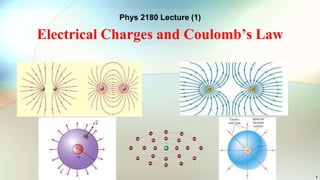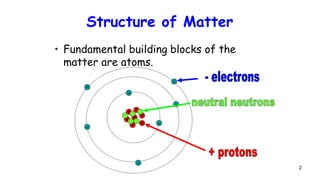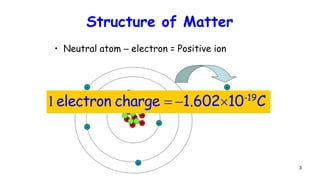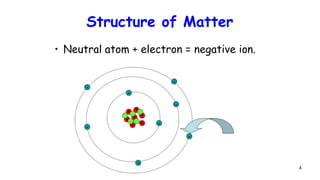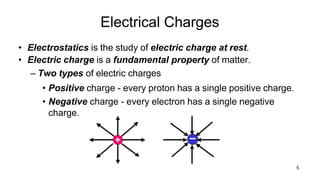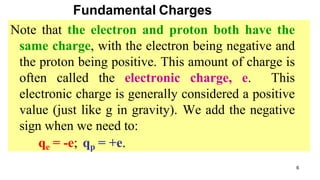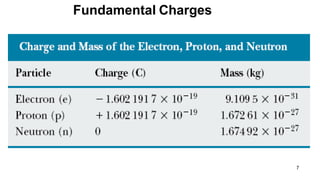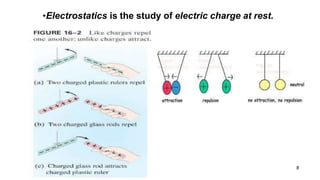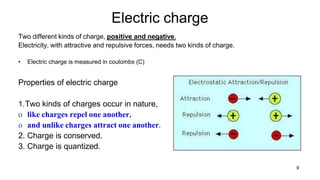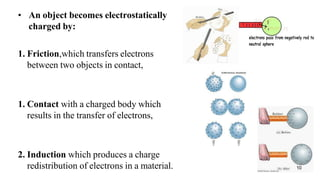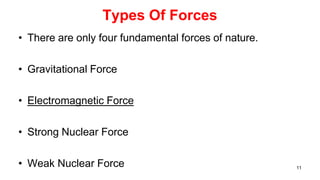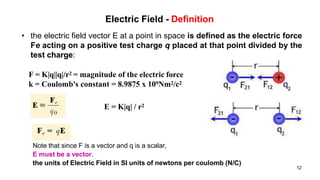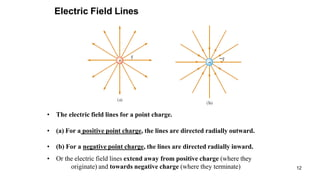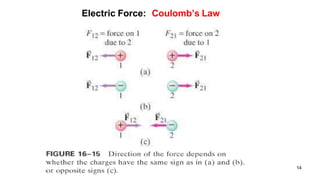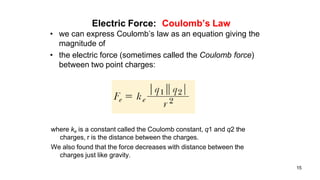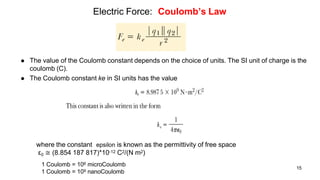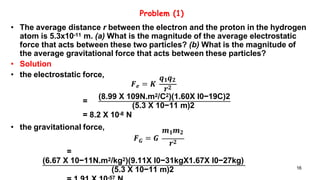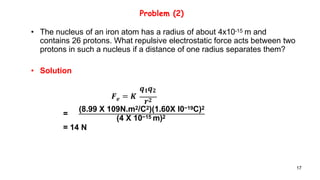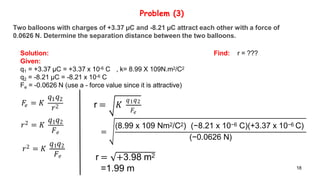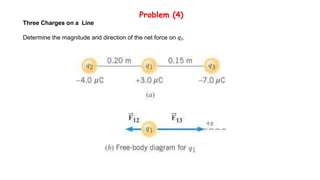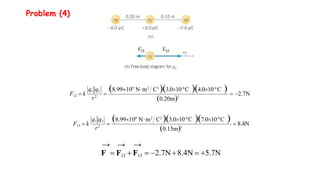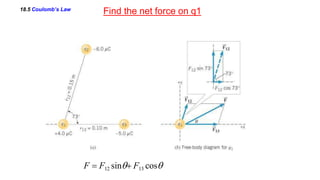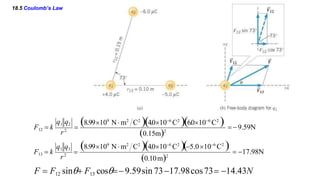1 von 23
Anzeige

### Electrical Charges and Coulomb's Law.pptx

1. Phys 2180 Lecture (1) Electrical Charges and Coulomb’s Law 1
2. Structure of Matter + + + + + + + - - • Fundamental building blocks of the matter are atoms. - - - - - 2
3. Structure of Matter • Neutral atom – electron = Positive ion + + + + + - - - + + - - - - 3 1electron charge  1.60210-19 C
4. Structure of Matter • Neutral atom + electron = negative ion. + - 4 + + + + + + - - - - - - -
5. Electrical Charges 5 • Electrostatics is the study of electric charge at rest. • Electric charge is a fundamental property of matter. – Two types of electric charges • Positive charge - every proton has a single positive charge. • Negative charge - every electron has a single negative charge.
6. Fundamental Charges Note that the electron and proton both have the same charge, with the electron being negative and the proton being positive. This amount of charge is often called the electronic charge, e. This electronic charge is generally considered a positive value (just like g in gravity). We add the negative sign when we need to: qe = -e; qp = +e. 6
7. Fundamental Charges 7
8. •Electrostatics is the study of electric charge at rest. 8
9. Electric charge Two different kinds of charge, positive and negative. Electricity, with attractive and repulsive forces, needs two kinds of charge. • Electric charge is measured in coulombs (C) Properties of electric charge 1.Two kinds of charges occur in nature, o like charges repel one another, o and unlike charges attract one another. 2. Charge is conserved. 3. Charge is quantized. 9
10. • An object becomes electrostatically charged by: 1. Friction,which transfers electrons between two objects in contact, 1. Contact with a charged body which results in the transfer of electrons, 2. Induction which produces a charge redistribution of electrons in a material. 10
11. 11 Types Of Forces • There are only four fundamental forces of nature. • Gravitational Force • Electromagnetic Force • Strong Nuclear Force • Weak Nuclear Force
12. Electric Field - Definition Note that since F is a vector and q is a scalar, E must be a vector. the units of Electric Field in SI units of newtons per coulomb (N/C) • the electric field vector E at a point in space is defined as the electric force Fe acting on a positive test charge q placed at that point divided by the test charge: F = K|q||q|/r2 = magnitude of the electric force k = Coulomb's constant = 8.9875 x 109Nm2/c2 E = K|q| / r2 12
13. Electric Field Lines • The electric field lines for a point charge. • (a) For a positive point charge, the lines are directed radially outward. • (b) For a negative point charge, the lines are directed radially inward. • Or the electric field lines extend away from positive charge (where they originate) and towards negative charge (where they terminate) 12
14. Electric Force: Coulomb’s Law 14
15. Electric Force: Coulomb’s Law • we can express Coulomb’s law as an equation giving the magnitude of • the electric force (sometimes called the Coulomb force) between two point charges: where ke is a constant called the Coulomb constant, q1 and q2 the charges, r is the distance between the charges. We also found that the force decreases with distance between the charges just like gravity. 15
16. Electric Force: Coulomb’s Law  The value of the Coulomb constant depends on the choice of units. The SI unit of charge is the coulomb (C).  The Coulomb constant ke in SI units has the value where the constant epsilon is known as the permittivity of free space ε0 ≅ (8.854 187 817)*10-12 C2/(N m2) 15 1 Coulomb = 106 microCoulomb 1 Coulomb = 109 nanoCoulomb
17. Problem (1) • The average distance r between the electron and the proton in the hydrogen atom is 5.3x10-11 m. (a) What is the magnitude of the average electrostatic force that acts between these two particles? (b) What is the magnitude of the average gravitational force that acts between these particles? • Solution • the electrostatic force, • the gravitational force, 16 𝑭𝒆 = 𝑲 𝒒𝟏𝒒𝟐 𝒓𝟐 = (8.99 X 109N.m2/C2)(1.60X l0−19C)2 (5.3 X 10−11 m)2 = 8.2 X 10-8 N 𝑮 𝒎𝟏𝒎𝟐 𝑭 = 𝑮 𝒓𝟐 = (6.67 X 10−11N.m2/kg2)(9.11X l0−31kgX1.67X l0−27kg) (5.3 X 10−11 m)2 -57
18. • The nucleus of an iron atom has a radius of about 4x10-15 m and contains 26 protons. What repulsive electrostatic force acts between two protons in such a nucleus if a distance of one radius separates them? • Solution 17 Problem (2) 𝒆 𝒒𝟏𝒒𝟐 𝑭 = 𝑲 𝒓𝟐 = (8.99 X 109N.m2/C2)(1.60X l0−19C)2 (4 X 10−15 m)2 = 14 N
19. 18 Problem (3) Two balloons with charges of +3.37 µC and -8.21 µC attract each other with a force of 0.0626 N. Determine the separation distance between the two balloons. Solution: Given: q1 = +3.37 µC = +3.37 x 10-6 C , k= 8.99 X 109N.m2/C2 q2 = -8.21 µC = -8.21 x 10-6 C Fe = -0.0626 N (use a - force value since it is attractive) Find: r = ??? 𝑒 𝐹 = 𝐾 𝑞 𝑞 1 2 𝑟2 𝑟2 = 𝐾 𝑞1𝑞2 𝐹𝑒 𝑟2 = 𝐾 𝑞1𝑞2 𝐹𝑒 r = 𝐾 𝑞1𝑞2 𝐹𝑒 = (8.99 x 109 Nm2/C2) (−8.21 x 10−6 C)(+3.37 x 10−6 C) (−0.0626 N) r = +3.98 m2 =1.99 m
20. Three Charges on a Line Determine the magnitude and direction of the net force on q1. Problem (4)
21. 8.99109 Nm2 C2 3.0106 C 4.0106 C  0.20m2  2.7N r2 q1 q2 F12  k  8.99109 Nm2 C2 3.0106 C 7.0106 C  0.15m2  8.4N r2 q1 q3 F13  k  → → → F  F12  F13  2.7N8.4N  5.7N Problem (4)
22. 18.5 Coulomb’s Law Find the net force on q1 F  F12 sin F13 cos
23. 18.5 Coulomb’s Law 0.15m2 8.99109 N m2 C2 4.0106 C2 6.0106 C2   9.59N q1 q2 F12  k  8.99109 N m2 C2 4.0106 C2 5.0106 C2  0.10m2  17.98N r2 r2 q1 q3 F13  k  F  F12 sin F13 cos9.59sin 7317.98cos73 14.43N
Anzeige